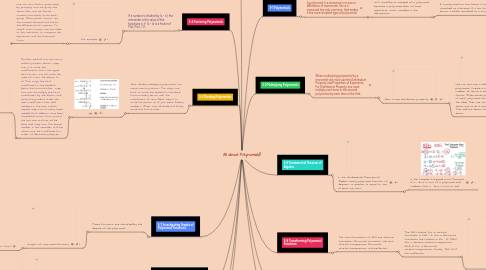Andrea Torres
Get Started. It's FreeAll about Polynomials!!## 1. 3-1 Polynomials

### 1.1. A polynomial is a monomial or a sum or difference of monomials. Since a monomial has only one term, that makes it the most simplest type of polynomial.

1.1.1. 4x^2 would be an example of a polynomial because a polynomial does not have exponents, roots, variables in the denominator.

1.1.1.1. If a polynomial has two terms it will be classified as a binomial. If it has three terms it will be classified as a trinomial.

1.1.1.1.1. Examples

## 2. 3-2 Multiplying Polynomials

### 2.1. When multiplying polynomial by a monomial one must use the Distributive Property and Properties of Exponents. For Distributive Property one must multiply each term in the second polynomial by each term in the first.

2.1.1. How to use distributive property

2.1.1.1. One can also use a table to multiply polynomials. Create a table by the number of terms in each polynomial factor. Afterwards put the terms of each polynomial along the sides of the table. Then one will multiply each terms and write it inside in the box. Then add the terms and combine like terms.

## 3. 3-3 Dividing Polynomials

### 3.1. When dividing multiplying polynomials one cause use long division. The steps are first to write the dividend in standard form including terms with the coefficient of zero. Next step is to write the division as if you were dividing numbers. After one will divide and finally write the final answer.

3.1.1. Ex.

3.1.1.1. Another method one can use is called Synthetic division. Step one is to write the coefficients and in the upper left corner, one will write the value of a for the divisor (x - a). Then copy the first coefficient in the dividend below the horizontal bar. Step two one will multiply the first coefficient by the divisor, and write the product under the next coefficient, then add numbers in the new column. Repeat step two as many times needed till all additions have been completed. Draw a box around the last sum and one will be done with step two. The boxed number is the reminder and the others are the coefficients in order of decreasing degree.

3.1.1.1.1. Example

## 4. 3-4 Factoring Polynomials

### 4.1. If a number is divided by (x - a), the remainder is the value of the functions a, if (x - a) is a factor of P(x), P(a) = 0.

4.1.1. For example

4.1.1.1. One can also factor polynomials by grouping. One will group the terms then one will factor common monomials from each group. Afterwards, factor out the common binomial and factor the difference of squares. To check one's answer use the table on the calculator to compare the expression and the factored form.

4.1.1.1.1. For example

## 5. 3-5 Finding Real Roots of Polynomial Equation

### 5.1. Factoring a polynomial equation can be a way to find it's real roots. One can find the solution or roots by using P(x) = 0, set each factor equal to zero and solve for the x.

5.1.1. Depending on the equation, if it is a quadratic, one will first factor out the greatest common factor then factor the quadratic. Subsequently, set each factor equal to zero then solve for x.

5.1.1.1. For example

## 6. 3-6 Fundamental Theorem of Algebra

### 6.1. In the Fundamental Theorem of Algebra every polynomial function of degree n is greater or equal to 1 has at least one zero.

6.1.1. In the Complex Conjugate Root Theorem, if a + bi is a root of a polynomial with numbers then a - bi is a root as well.

## 8. 3-8 Transforming Polynomial Functions

### 8.1. The transformations of f(x) are Vertical translation, Horizontal translation, Vertical stretch/compression, Horizontal stretch/compression, and Reflection.

8.1.1. The f(x) notation for a Vertical translation is f(x) + k. For a Horizontal translation the notation is f(x - h). Af(x) for a Vertical stretch/compression. F(1/b x) for a Horizontal stretch/compression. Lastly, -f(x), f(-x) for Reflection.

8.1.1.1. .

## 9. Vocabulary 3-1

### 9.1. Monomial - a number/product of numbers and variables with whole number exponents.

9.1.1. Polynomial - a monomial or a sum or difference of monomials.

9.1.1.1. Degree of a monomial - the sum of the exponents of the variables.

9.1.1.1.1. Degree of a polynomial - the degree of the first term indicates it.

## 10. Vocabulary 3-7

### 10.1. End behavior - a description of the values of the function as x approaches positive infinity (x is + infinity) or negative infinity ( x is - infinity).

10.1.1. Turning point - where a graph changes from increasing to decreasing or from decreasing to increasing.

10.1.1.1. Local maximum - if there is an interval around a such that f(x) less than f(a) for every x-value in the interval except a.

10.1.1.1.1. Local minimum - if there is an interval around a such that f(x) is greater than f(a) for every x-value in the interval except a.

## 11. 3-9 Curve Fitting with Polynomial Models

### 11.1. To make a mathematical model for a data given by a table, one will need to figure out which function will be appropriate.

11.1.1. Finite Differences can help one identify the degree of any polynomial data.

11.1.1.1. Something to help one find the degree

11.1.1.1.1. Example

## 12. 3-7 Investigating Graphs of Polynomial Functions

### 12.1. These functions are classified by the degree of the polynomial.

12.1.1. Graphs of Polynomial Functions

12.1.1.1. Polynomial End Behavior Chart

12.1.1.1.1. Ex. P(x) = -4x^4 - 3x^2 + 5x + 6, The leading coefficient is -4, The degree is 3, which is odd., As x is - infinity, P(x) is + infinity, and x is + infinity, P(x) is - infinity.